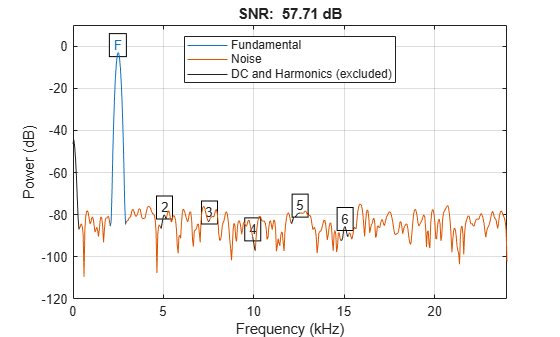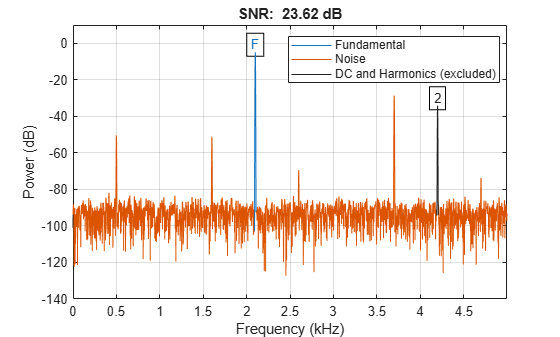# snr

Signal-to-noise ratio

## Syntax

``r = snr(xi,y)``
``r = snr(x)``
``r = snr(x,fs,n)``
``r = snr(pxx,f,'psd')``
``r = snr(pxx,f,n,'psd')``
``r = snr(sxx,f,rbw,'power')``
``r = snr(sxx,f,rbw,n,'power')``
``r = snr(___,'aliased')``
``````[r,noisepow] = snr(___)``````
``snr(___)``

## Description

example

````r = snr(xi,y)` returns the signal-to-noise ratio (SNR) in decibels of a signal, `xi`, by computing the ratio of its summed squared magnitude to that of the noise `y`: ```r = mag2db(rssq(xi(:))/rssq(y(:)))```.`y` must have the same dimensions as `xi`. Use this form when the input signal is not necessarily sinusoidal and you have an estimate of the noise.```

example

````r = snr(x)` returns the SNR in decibels relative to the carrier (dBc) of a real-valued sinusoidal input signal, `x`. The SNR is determined using a modified periodogram of the same length as the input. The modified periodogram uses a Kaiser window with β = 38. The result excludes the power of the first six harmonics, including the fundamental.```

example

````r = snr(x,fs,n)` returns the SNR in dBc of a real sinusoidal input signal, `x`, sampled at a rate `fs`. The computation excludes the power contained in the lowest `n` harmonics, including the fundamental. The default value of `fs` is 1. The default value of `n` is 6.```

example

````r = snr(pxx,f,'psd')` specifies the input `pxx` as a one-sided power spectral density (PSD) estimate. The argument `f` is a vector of the frequencies at which the estimates of `pxx` occur. The computation of noise excludes the power of the first six harmonics, including the fundamental.```
````r = snr(pxx,f,n,'psd')` specifies the number of harmonics, `n`, to exclude when computing the SNR. The default value of `n` is 6 and includes the fundamental.```

example

````r = snr(sxx,f,rbw,'power')` specifies the input as a one-sided power spectrum, `sxx`, of a real signal. The input `rbw` is the resolution bandwidth over which each power estimate is integrated.```
````r = snr(sxx,f,rbw,n,'power')` specifies the number of harmonics, `n`, to exclude when computing the SNR. The default value of `n` is 6 and includes the fundamental.```

example

````r = snr(___,'aliased')` removes harmonics of the fundamental that are aliased into the Nyquist range. Use this option when the sinusoidal input signal is undersampled. If you do not specify this option, or if you set it to `'omitaliases'`, then the function treats as noise any harmonics of the fundamental frequency that lie beyond the Nyquist range.```

example

``````[r,noisepow] = snr(___)``` also returns the total noise power of the nonharmonic components of the signal.```

example

````snr(___)` with no output arguments plots the spectrum of the signal in the current figure window and labels its main features. It uses different colors to draw the fundamental component, the DC value and the harmonics, and the noise. The SNR appears above the plot. This functionality works for all syntaxes listed above except `snr(x,y)`.```

## Examples

collapse all

Generate a 20-millisecond rectangular pulse sampled for 2 seconds at 10 kHz.

```Tpulse = 20e-3; Fs = 10e3; t = -1:1/Fs:1; x = rectpuls(t,Tpulse);```

Embed the pulse in white Gaussian noise such that the signal-to-noise ratio (SNR) is 53 dB. Reset the random number generator for reproducible results.

```rng default SNR = 53; y = randn(size(x))*std(x)/db2mag(SNR); s = x + y;```

Use the `snr` function to compute the SNR of the noisy signal.

`pulseSNR = snr(x,y)`
```pulseSNR = 53.1255 ```

Compute and compare the signal-to-noise ratio (SNR), the total harmonic distortion (THD), and the signal to noise and distortion ratio (SINAD) of a signal.

Create a sinusoidal signal sampled at 48 kHz. The signal has a fundamental of frequency 1 kHz and unit amplitude. It additionally contains a 2 kHz harmonic with half the amplitude and additive noise with variance 0.1².

```fs = 48e3; t = 0:1/fs:1-1/fs; A = 1.0; powfund = A^2/2; a = 0.4; powharm = a^2/2; s = 0.1; varnoise = s^2; x = A*cos(2*pi*1000*t) + ... a*sin(2*pi*2000*t) + s*randn(size(t));```

Verify that SNR, THD, and SINAD agree with their definitions.

```SNR = snr(x); defSNR = 10*log10(powfund/varnoise); SN = [SNR defSNR]```
```SN = 1×2 17.0178 16.9897 ```
```THD = thd(x); defTHD = 10*log10(powharm/powfund); TH = [THD defTHD]```
```TH = 1×2 -7.9546 -7.9588 ```
```SINAD = sinad(x); defSINAD = 10*log10(powfund/(powharm+varnoise)); SI = [SINAD defSINAD]```
```SI = 1×2 7.4571 7.4473 ```

Compute the SNR of a 2.5 kHz sinusoid sampled at 48 kHz. Add white noise with variance 0.001².

```Fi = 2500; Fs = 48e3; N = 1024; x = sin(2*pi*Fi/Fs*(1:N)) + 0.001*randn(1,N); SNR = snr(x,Fs)```
```SNR = 57.7103 ```

Plot the spectrum and annotate the SNR.

`snr(x,Fs);`Obtain the periodogram power spectral density (PSD) estimate of a 2.5 kHz sinusoid sampled at 48 kHz. Add white noise with standard deviation 0.00001. Use this value as input to determine the SNR. Set the random number generator to the default settings for reproducible results.

```rng default Fi = 2500; Fs = 48e3; N = 1024; x = sin(2*pi*Fi/Fs*(1:N)) + 0.00001*randn(1,N); w = kaiser(numel(x),38); [Pxx, F] = periodogram(x,w,numel(x),Fs); SNR = snr(Pxx,F,'psd')```
```SNR = 97.7446 ```

Using the power spectrum, compute the SNR of a 2.5 kHz sinusoid sampled at 48 kHz and embedded in white noise with a standard deviation of 0.00001. Reset the random number generator for reproducible results.

```rng default Fi = 2500; Fs = 48e3; N = 1024; x = sin(2*pi*Fi/Fs*(1:N)) + 0.00001*randn(1,N); w = kaiser(numel(x),38); [Sxx, F] = periodogram(x,w,numel(x),Fs,'power'); rbw = enbw(w,Fs); SNR = snr(Sxx,F,rbw,'power')```
```SNR = 97.7446 ```

Plot the spectrum of the signal and annotate the SNR.

`snr(Sxx,F,rbw,'power');`Generate a signal that resembles the output of a weakly nonlinear amplifier with a 2.1 kHz tone as input. The signal is sampled for 1 second at 10 kHz. Compute and plot the power spectrum of the signal. Use a Kaiser window with β = 38 for the computation.

```Fs = 10000; f = 2100; t = 0:1/Fs:1; x = tanh(sin(2*pi*f*t)+0.1) + 0.001*randn(1,length(t)); periodogram(x,kaiser(length(x),38),[],Fs,'power')```Harmonics stick out from the noise at frequencies of 4.2 kHz, 6.3 kHz, 8.4 kHz, 10.5 kHz, 12.6 kHz, and 14.7 kHz. All frequencies except for the first one are greater than the Nyquist frequency. The harmonics are aliased respectively into 3.7 kHz, 1.6 kHz, 0.5 kHz, 2.6 kHz, and 4.7 kHz.

Compute the signal-to-noise ratio of the signal. By default, `snr` treats the aliased harmonics as part of the noise.

`snr(x,Fs,7);`Repeat the computation, but now treat the aliased harmonics as part of the signal.

`snr(x,Fs,7,'aliased');`Create a sinusoidal signal sampled at 48 kHz. The signal has a fundamental of frequency 1 kHz and unit amplitude. It additionally contains a 2 kHz harmonic with half the amplitude and additive noise with variance 0.1².

```fs = 48e3; t = 0:1/fs:1-1/fs; A = 1.0; powfund = A^2/2; a = 0.4; powharm = a^2/2; s = 0.1; varnoise = s^2; x = A*cos(2*pi*1000*t) + ... a*sin(2*pi*2000*t) + s*randn(size(t));```

Compute the noise power in the signal. Verify that it agrees with the definition.

```[SNR,npow] = snr(x,fs); compare = [10*log10(powfund)-npow SNR]```
```compare = 1×2 17.0281 17.0178 ```

Generate a sinusoid of frequency 2.5 kHz sampled at 50 kHz. Reset the random number generator. Add Gaussian white noise with standard deviation 0.00005 to the signal. Pass the result through a weakly nonlinear amplifier. Plot the SNR.

```rng default fs = 5e4; f0 = 2.5e3; N = 1024; t = (0:N-1)/fs; ct = cos(2*pi*f0*t); cd = ct + 0.00005*randn(size(ct)); amp = [1e-5 5e-6 -1e-3 6e-5 1 25e-3]; sgn = polyval(amp,cd); snr(sgn,fs);```The DC component and all harmonics, including the fundamental, are excluded from the noise measurement. The fundamental and harmonics are labeled.

## Input Arguments

collapse all

Input signal, specified as a vector, matrix, or N-D array.

Data Types: `double` | `single`
Complex Number Support: Yes

Estimate of the noise in the input signal, specified as a vector, matrix, or N-D array with the same dimensions as `xi`.

Data Types: `double` | `single`
Complex Number Support: Yes

Real-valued sinusoidal input signal, specified as a row or column vector.

Data Types: `double` | `single`

Sample rate, specified as a positive scalar. The sample rate is the number of samples per unit time. If the unit of time is seconds, then the sample rate has units of hertz.

Data Types: `double` | `single`

Number of harmonics to exclude from the SNR computation, specified as a positive integer scalar. The default value of `n` is 6.

One-sided power spectral density estimate, specified as a real-valued, nonnegative column vector.

The power spectral density must be expressed in linear units, not decibels. Use `db2pow` to convert decibel values to power values.

Example: ```[pxx,f] = periodogram(cos(pi./[4;2]*(0:159))'+randn(160,2))``` specifies the periodogram PSD estimate of a noisy two-channel sinusoid sampled at 2π Hz and the frequencies at which it is computed.

Data Types: `double` | `single`

Cyclical frequencies of the one-sided PSD estimate, `pxx`, specified as a row or column vector. The first element of `f` must be 0.

Data Types: `double` | `single`

Power spectrum, specified as a real-valued nonnegative row or column vector.

The power spectrum must be expressed in linear units, not decibels. Use `db2pow` to convert decibel values to power values.

Example: ```[sxx,w] = periodogram(cos(pi./[4;2]*(0:159))'+randn(160,2),'power')``` specifies the periodogram power spectrum estimate of a two-channel sinusoid embedded in white Gaussian noise and the normalized frequencies at which it is computed.

Data Types: `double` | `single`

Resolution bandwidth, specified as a positive scalar. The resolution bandwidth is the product of the frequency resolution of the discrete Fourier transform and the equivalent noise bandwidth of the window.

Data Types: `double` | `single`

## Output Arguments

collapse all

Signal-to-noise ratio, expressed in decibels relative to the carrier (dBc), returned as a real-valued scalar. The SNR is returned in decibels (dB) if the input signal is not sinusoidal.

Data Types: `double` | `single`

Total noise power of the nonharmonic components of the input signal, returned as a real-valued scalar.

Data Types: `double` | `single`

collapse all

### Distortion Measurement Functions

The functions `thd`, `sfdr`, `sinad`, and `snr` measure the response of a weakly nonlinear system stimulated by a sinusoid.

When given time-domain input, `snr` performs a periodogram using a Kaiser window with large sidelobe attenuation. To find the fundamental frequency, the algorithm searches the periodogram for the largest nonzero spectral component. It then computes the central moment of all adjacent bins that decrease monotonically away from the maximum. To be detectable, the fundamental should be at least in the second frequency bin. Higher harmonics are at integer multiples of the fundamental frequency. If a harmonic lies within the monotonically decreasing region in the neighborhood of another, its power is considered to belong to the larger harmonic. This larger harmonic may or may not be the fundamental.

The function estimates a noise level using the median power in the regions containing only noise. The DC component is excluded from the calculation. The noise at each point is the estimated level or the ordinate of the point, whichever is smaller. The noise is then subtracted from the values of the signal and the harmonics.

`snr` fails if the fundamental is not the highest spectral component in the signal.

Ensure that the frequency components are far enough apart to accommodate for the sidelobe width of the Kaiser window. If this is not feasible, you can use the `'power'` flag and compute a periodogram with a different window.

## Version History

Introduced in R2013b